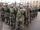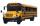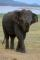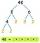The balls

You have 108 red and 180 green balls. You have to be grouped into the bags so that the ratio of red to green in each bag was the same. What smallest number of balls may be in one bag?

Result

n =  8

Solution:Leave us a comment of example and its solution (i.e. if it is still somewhat unclear...):Be the first to comment!To solve this example are needed these knowledge from mathematics:

Need help calculate sum, simplify or multiply fractions? Try our fraction calculator.

Next similar examples:

1. TroopsIf the general sorts troops into the crowd by nine left 6. How many soldiers has regiment if we know that they are less than 300?
2. Reminder and quotientThere are given the number C = 281, D = 201. Find the highest natural number S so that the C:S, D:S are with the remainder of 1,
3. Energy savingThey were released three different, independent inventions saving 12%, 15% and 25% energy. Some considered that while the use of these inventions, the total savings will be 12% + 15% + 25% = 52%. Is this true? How much percent of energy will save all thre
4. Watching TVOne evening 2/3 students watch TV. Of those students, 3/8 watched a reality show. Of the students that watched the show, 1/4 of them recorded it. What fraction of the students watched and recorded reality tv.
5. DivisibilityWrite all the integers x divisible by seven and eight at the same time for which the following applies: 100
6. PipeSteel pipe has a length 2.5 meters. About how many decimetres is 1/3 less than 4/8 of this steel pipe?
7. LinesFive bus lines runs at intervals 3, 6, 9, 12, 15 minutes. In the morning, suddenly start at 4:00. After how many minutes the bus lines meet again?
8. EqnSolve equation with fractions: 2x/3-50=40+x/4
9. 100 %How much is 100%, if 17 % is 1169?
10. TeacherTeacher Rem bought 360 pieces of cupcakes for the outreach program of their school. 5/9 of the cupcakes were chocolate flavor and 1/4 wete pandan flavor and the rest were vanilla flavor. How much more chocolate flavor cupcakes than vanilla flavor?
11. Write 2Write 791 thousandths as fraction in expanded form.
12. KingKing had four sons. First inherit 1/2, second 1/4 , third 1/5 of property. What part of the property was left to the last of the brothers?
13. Fractions 4How many 2/3s are in 6?
14. DecompositionMake decomposition using prime numbers of number 155. Result write as prime factors (all, even multiple)
15. PearsThere were pears in the basket, I took two-fifths of them, and left six in the basket. How many pears did I take?
16. Pizza 4Marcus ate half pizza on monday night. He than ate one third of the remaining pizza on Tuesday. Which of the following expressions show how much pizza marcus ate in total?
17. Unknown numberI think the number - its sixth is 3 smaller than its third.